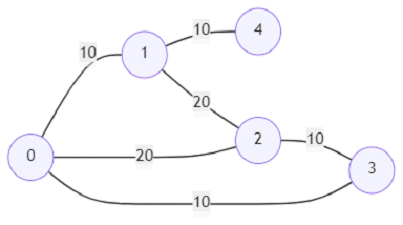# Program to find out if a vertex in an undirected graph has a lesser cost path in Python

Suppose, we are given a weighted, undirected graph. We have to implement a function query that takes two vertices and a cost 'limit' as input and checks if there exists a lower cost path than the cost given as input. We return true if there exists a path or otherwise, we return false.

So, if the input is likeand the queries are (0, 2, 10), (3, 1, 30), (4, 3, 30).

then the output will be

False
True
True

The result of the first query is False because there is no path between vertex 0 to 2 of cost 10.

The result of the second query is True because there is a path between vertex 3 to 1 of cost 10, that is less than 30.

The result of the third query is True because there is a path between vertex 4 to 3 of cost 30, that is equal to 30.

To solve this, we will follow these steps −

• weights := a list containing the different weights in the graph

• connections := a list containing the connections for the weights

• Define the function query() . This will take p, q, limit

• index := the position in weights here limit can be inserted to the left of maintaining the sorted order

• if index is same as 0, then

• return False

• return True if connections[index-1, p] is same as connections[index-1, q]

## Example

Let us see the following implementation to get better understanding −

import bisect
class Solution(object):

def __init__(self, n, edgeList):
def find(node):
if parent[node]!=node:
parent[node] = find(parent[node])
return parent[node]

def union(x,y):
parent[find(y)] = find(x)
return

parent = {i:i for i in range(n)}
edgeList.sort(key = lambda x:x)
self.connections = []
self.weights = []
for index,(i,j,weight) in enumerate(edgeList):
union(i,j)
if index!=len(edgeList)-1 and weight == edgeList[index+1]:
continue
self.weights.append(weight)
self.connections.append([find(i) for i in parent])

def query(self, p, q, limit):
index = bisect.bisect_left(self.weights,limit)
if index==0:
return False
return self.connections[index-1][p] == self.connections[index-1][q]

ob = Solution(5, [[0, 1, 10], [0, 2, 20], [1, 4, 10], [0, 3, 10], [1, 2, 20], [2, 3, 10]])
print(ob.query(0, 2, 10))
print(ob.query(3, 1, 30))
print(ob.query(4, 3, 30))

## Input

ob = Solution(5, [[0, 1, 10], [0, 2, 20], [1, 4, 10], [0, 3, 10], [1, 2, 20], [2, 3, 10]])
print(ob.query(0, 2, 10))
print(ob.query(3, 1, 30))
print(ob.query(4, 3, 30))

## Output

False
True
True

Updated on: 08-Oct-2021

89 Views